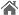您的当前位置: 首页 > 产品中心 > 电动消防泵组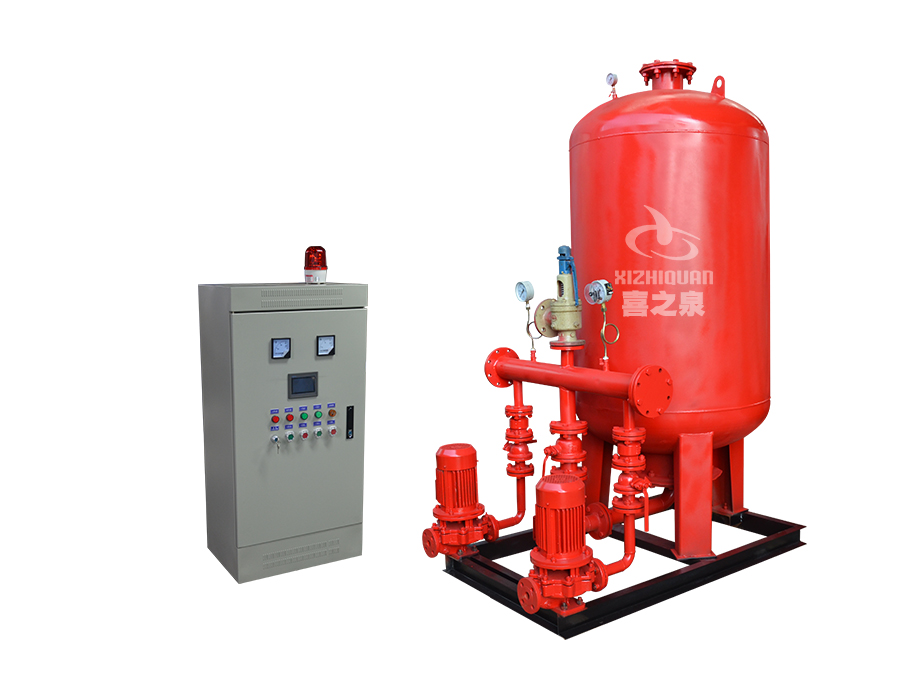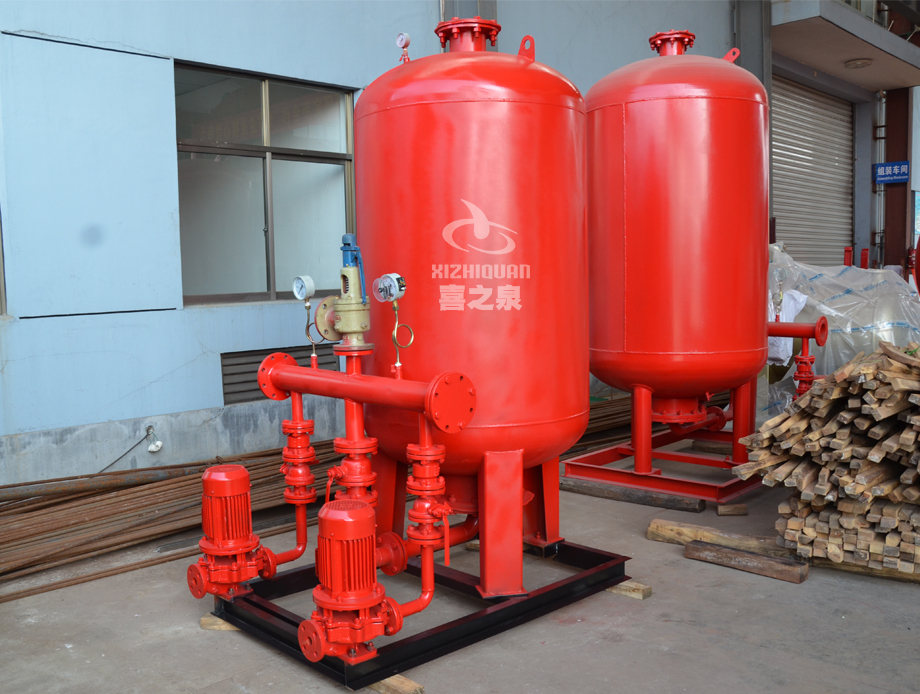# XZQ上置式消防稳压给水设备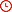2019-07-20XZQ上置式消防稳压给水设备，消防自动增压稳压给水成套设备是为消防灭火工程配套的设备，主要作用：保持水灭火管网的消防压力，发生火警打开水灭火设备能立即喷出充实水柱

• 流量： 1～5（m3/h）
• 扬程： 30-150（m）
• 功率： 0.75-15（kw）
• 转速： 2900（r/min）

【 产 品 概 况 】

消防自动增压稳压给水成套设备是为消防灭火工程配套的设备，主要作用：保持水灭火管网的消防压力，发生火警打开水灭火设备能立即喷出充实水柱，给报警联动启动大消防泵赢得30秒以上的初期灭火扑救时间，直至消防主泵全负荷启动进行，由于该设备能始终保持管网压力，使管网不存空气启动大消防泵不打呛管网无颤动危险。并获得消防产品3CF认证证书。

【产品结构】

该设备由稳压泵两台(根据设计要求可设一台)为一用一备，隔膜式稳压罐一台，电控柜一台，仪表阀门及组合管网各一套，组成了隔膜式稳压供水设备。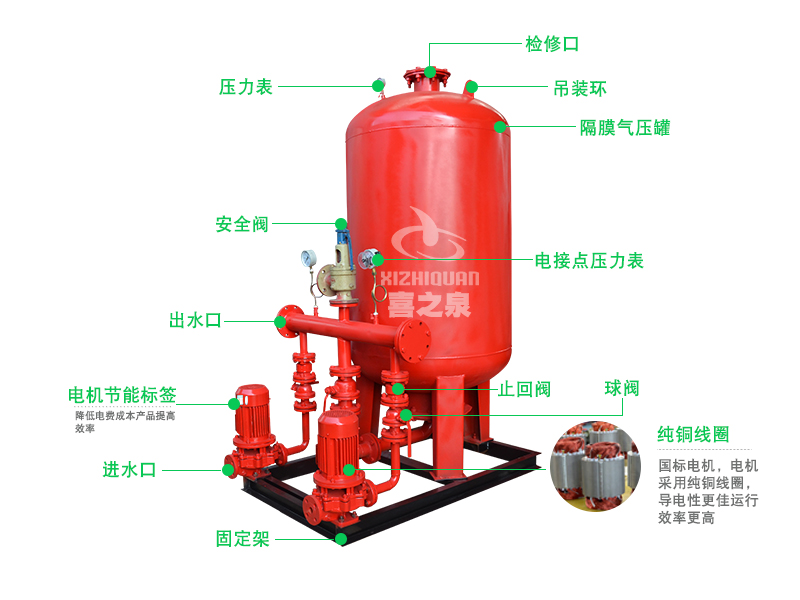【产品特点】

1、泵、电控柜、隔膜式气压罐、组合管网四位一体，并配带有隔振器，无需预埋地脚孔，设计人员只需算好参数即可查得设备型号、基础尺寸等。电气设计人员无需再进行控制线路设计，只设计泵房内进线和到设备的走线。选用方便，可大大缩短设计人员的设计周期和施工人员的施工时间。

2、隔膜式气压罐一次性充气持久耐用，水气隔离能预防水质的污染。

3、设备占地面积小，投资省，全自动运行，无需专人看管。

4、设备有双电源接口，双路电源自动(手动)切换,设备可根据设计要求制作。

5、具有自动保护及故障切换功能。任何一台水泵发生故障(电气故障或水力故障)均能启动备用泵。

6、可与消防 连接(根据设计需要)。

7、可以根据客户的需要选择罐、稳压方式、控制功能。

8、电气主要元气件采用国产或企业产品，质量可靠、运行稳定

【 选 择 型 号 】

ZW(L、W)本设备根据建设部标准图集98S205基础的性能参数做为参考，推出了两种设备型号，①ZW(L)、ZW(W)系列稳压按照建设部图集98S205图集号所设计的型号;②企业根据消防GA30-92、GA30-2002相关标准及给水设备标准规范编制的设备型号。在这里删除了稳压罐、水泵的垄断性型号，给设计及用户一个产品选择的竞争空间。本公司唐纯虎为您推荐以下型号产品使用，如：LG、GDL、CDL、ISG等型号。用户可以根据水位水箱间的位置选择合适的设备。

【 工 作 原 理 】

隔膜式气压给水设备，一次充气，常年运行使用。工作时起动补水泵，水室进水，水压升高，气室的气体被压缩隔膜伸长;当水压下降时，气室气体膨胀，隔膜收缩，压迫水室出水。这样周而复始，通过电接点压力表和电控箱控制水泵运行，达到额定的压力和连续供水。

【 产 品 用 途 】

1、工业、民用建筑的消防、喷淋和稳压给水;

2、暖通、空调、锅炉的定压补水;

3、隐蔽工程、临时建筑和小型工矿，偏远地区的生活给水

【 性 能 参 数 】

 序号 增压稳压设备型号 消防压力(Mpa)P1 立式隔膜式气压罐 配用水泵 运行压力 稳压水容积(L) 型号规格 工作压力比αb 消防储水容积(L) 型 号 标定容积 实际容积 (Mpa) 1 ZW(L)-1-X-7 0.1 SQL800X0.6 0.6 300 319 25LG3-10X4   N=1.5kw P1=0.10 PS1=0.26 54 P1=0.23 PS2=0.31 2 ZW(L)-1-Z-10 0.16 SQL800X0.6 0.8 150 159 25LG3-10X4   N=1.5kw P1=0.16 PS1=0.26 70 P1=0.23 PS2=0.36 3 ZW(L)-1-X-10 0.16 SQL800X0.6 0.6 300 319 25LG3-10X5   N=1.5kw P1=0.16 PS1=0.36 52 P1=0.33 PS2=0.42 4 ZW(L)-1-X-13 0.22 SQL1000X0.6 0.76 300 329 25LG3-10X4   N=1.5kw P1=0.22 PS1=0.35 97 P1=0.32 PS2=0.40 5 ZW(L)-1-XZ-10 0.16 SQL1000X0.6 0.65 450 480 25LG3-10X4   N=1.5kw P1=0.16 PS1=0.33 86 P1=0.30 PS2=0.38 6 ZW(L)--XZ-13 0.22 SQL1000X0.6 0.67 450 452 25LG3-10X5   N=1.5kw P1=0.22 PS1=0.41 80 P1=0.38 PS2=0.46 7 ZW(L)-II-Z- A 0.22 -0.38 SQL800X0.6 0.8 150 159 25LG3-10X6   N=2.2kw P1=0.38 PS1=0.53 61 P1=0.50 PS2=0.60 8 B 0.38 -0.50 SQL800X1.0 0.8 150 159 25LG3-10X8   N=2.2kw P1=0.50 PS1=0.68 51 P1=0.65 PS2=0.75 9 C 0.50 -0.65 SQL1000X1.5 0.85 150 206 25LG3-10X9   N=2.2kw P1=0.65 PS1=0.81 59 P1=0.78 PS2=0.86 10 D 0.65 -0.85 SQL1000X1.5 0.85 150 206 25LG3-10X11   N=3.0kw P1=0.85 PS1=1.04 57 P1=1.02 PS2=1.10 11 E 0.85 -1.0 SQL1000X1.5 0.85 150 206 25LG3-10X13   N=4.0kw P1=1.00 PS1=1.21 50 P1=1.19 PS2=1.27 12 ZW(L)-II-X- A 0.22-0.38 SQL1000X0.6 0.78 300 302 25LG3-10X6   N=2.2kw P1=0.38 PS1=0.53 72 P1=0.50 PS2=0.60 13 B 0.38-0.50 SQL1000X1.0 0.78 300 302 25LGW3-10X8   N=2.2kw P1=0.50 PS1=0.68 61 P1=0.65 PS2=0.75 14 C 0.50-0.65 SQL1000X1.5 0.78 300 302 25LG3-10X10   N=3.0kw P1=0.65 PS1=0.88 51 P1=0.86 PS2=0.93 15 D 0.65-0.85 SQL1200X1.5 0.85 300 355 25LG3-10X13   N=4.0kw P1=0.85 PS1=1.05 82 P1=1.02 PS2=1.10 16 E 0.85-1.0 SQL1200X1.5 0.85 300 355 25LG3-10X15   N=4.0kw P1=1.00 PS1=1.21 73 P1=1.19 PS2=1.26 17 ZW(L)-II-XZ- A 0.22-0.38 SQL1200X0.6 0.8 450 474 25LG3-10X6   N=2.2kw P1=0.38 PS1=0.53 133 P1=0.50 PS2=0.60 18 B 0.38-0.50 SQL1200X1.0 0.8 450 474 25LG3-10X8   N=2.2kw P1=0.50 PS1=0.68 110 P1=0.65 PS2=0.75 19 C 0.50-0.65 SQL1200X1.5 0.8 450 474 25LG3-10X10   N=3.0kw P1=0.65 PS1=0.81 90 P1=0.78 PS2=0.86 20 D 0.65-0.85 SQL1200X1.5 0.8 450 474 25LG3-10X12   N=4.0kw P1=0.85 PS1=1.04 73 P1=1.02 PS2=1.10 21 E 0.85-1.0 SQL1200X1.5 0.8 450 474 25LG3-10X14   N=4.0kw P1=1.00 PS1=1.21 64 P1=1.19 PS2=1.27